2017-03-29 22:53:23 gxupter 阅读数 2130
• ###### 单片机控制第一个外设-LED灯-第1季第6部分

本课程是《朱有鹏老师单片机完全学习系列课程》第1季第6个课程，主要讲解LED的工作原理和开发板原理图、实践编程等，通过学习目的是让大家学会给单片机编程控制LED灯，并且为进一步学习其他外设打好基础。

4000 人正在学习 去看看 朱有鹏2019-07-12 14:08:38 qq_36369267 阅读数 15
• ###### 单片机控制第一个外设-LED灯-第1季第6部分

本课程是《朱有鹏老师单片机完全学习系列课程》第1季第6个课程，主要讲解LED的工作原理和开发板原理图、实践编程等，通过学习目的是让大家学会给单片机编程控制LED灯，并且为进一步学习其他外设打好基础。

4000 人正在学习 去看看 朱有鹏

(1)运行程序，打开数据窗口，观察地址 30H、31H、32H、33H的数据变化。用键盘输入改变地址 30H、31H、32H、33H 的

(2)打开 CPU 窗口，选择单步或跟踪执行方式运行程序，观察 CPU 窗口各寄存器的变化。
(3)教材第 55 页 5、7、8 题。

``````;实验2-1
ORG 0000H
LJMP START
FUNC0: MOV 30H,#6
RET
FUNC1: MOV 31H,#7
RET
FUNC2: MOV 32H,#8
RET
FUNC3: MOV 33H,#9
RET
FUNCENTER:
MOV DPTR,#FUNCTAB
JMP @A+DPTR
FUNCTAB:
AJMP FUNC0
AJMP FUNC1
AJMP FUNC2
AJMP FUNC3
START:
MOV A,#0
CALL FUNCENTER
MOV A,#1
CALL FUNCENTER
MOV A,#2
CALL FUNCENTER
MOV A,#3
CALL FUNCENTER
SJMP \$
END``````

``````MOV A,#56H

Cy=1, OV=0, A=94H

01010110

+01110100

————————

11001010  （第二条指令执行完，CY=0,CY-1=1, OV=CY⊕CY-1=1）

11001010

+11001010

————————

110010100（第三条指令执行完，CY=1,CY-1=1, OV=CY⊕CY-1=0）

``````MOV A,59H
MOV R0,A
MOV A,#0
MOV @R0,A
MOV A,#25H
MOV 51H,A
MOV 52H,#70H``````

A=25H, (50H)=0H, (51H)=25H, (52H)=70H

``````PUSH 30H  ;SP=(61H)     (SP)=(24H)
PUSH 31H  ;SP=(62H)     (SP)=(10H)
POP DPL    ;SP=(61H)      DPL=(10H)
POP DPH   ;SP=(60H)      DPH=(24H)
MOV A,#00H
MOVX @DPTR,A``````

2012-12-09 12:54:14 zhangxxxww 阅读数 2417
• ###### 单片机控制第一个外设-LED灯-第1季第6部分

本课程是《朱有鹏老师单片机完全学习系列课程》第1季第6个课程，主要讲解LED的工作原理和开发板原理图、实践编程等，通过学习目的是让大家学会给单片机编程控制LED灯，并且为进一步学习其他外设打好基础。

4000 人正在学习 去看看 朱有鹏

一开始接触单片机中断，或许大部分人不知道什么时候用的上，又该怎么样用。其实，中断的运用极大地提高了工作的效率，中断在自动化行业是时时刻刻都在用，就像我们的计算机，一开始按照内置程序运行，但是当我们敲打键盘或者是点击鼠标时，它就必须停下当前的工作来响应现在的工作。

学习中断，就必须了解中断的寄存器和中断的优先级及中断如何使用如何定义等一系列的问题。

一个简单的单片机中断初始化程序

TMOD=0x01；//工作方式选择，选用T0

设置初值

TH0=（65536-50000）/256;//高八位

TL0=(65536-50000)%256;//低八位

EA=1；//开总中断

ET0=1；//开定时器0中断

TR0=1；//启动定时器中断

2018-10-24 01:00:59 qq_42352666 阅读数 3661
• ###### 单片机控制第一个外设-LED灯-第1季第6部分

本课程是《朱有鹏老师单片机完全学习系列课程》第1季第6个课程，主要讲解LED的工作原理和开发板原理图、实践编程等，通过学习目的是让大家学会给单片机编程控制LED灯，并且为进一步学习其他外设打好基础。

4000 人正在学习 去看看 朱有鹏

# 认识

## 中断

``````/*!
*  @brief      main函数
*  @since      v50
*  @note       山.外摄像头 LCD 测试实验
*/
void  main(void)
{
DisableInterrupts;        //关闭总中断

PLL_Init(PLL200);         //初始化PLL为200M，总线为100MHZ   决定单片机处理速度
camera_init(imgbuff);       //摄像头初始化
UART_Init(UART_4,115200);   //蓝牙或串口初始化

FTM_PWM_Init(FTM3,FTM_CH1,312,250);//PTE8    //电机1-A
GPIO_Init(PTE,8,GPO,1);
FTM_PWM_Init(FTM3,FTM_CH5,312,250);//PTE12    //电机2-A
GPIO_Init(PTE,12,GPO,1);

FTM_PWM_Init(FTM0 ,FTM_CH1,62500,duty);//PTC2 50000-125HZ

EnableInterrupts;

while(1)
{
camera_get_img();                                   //摄像头获取图像
find_line();
SendPicture();//调试时  一定要注释这一行！！！
}
}

void SendPicture(void)
{
int i=0,j=0;
UART_Put_Char(UART_4,0x01);
UART_Put_Char(UART_4,0xFE);
for(i=0;i<OV7725_EAGLE_H;i++)
{
for(j=0;j<OV7725_EAGLE_W;j++)
{
UART_Put_Char(UART_4,Image_data[i][j]);
}
}
UART_Put_Char(UART_4,0xFE);
UART_Put_Char(UART_4,0x01);
}

``````

## 串口

### 蓝牙串口

1、无线蓝牙串口：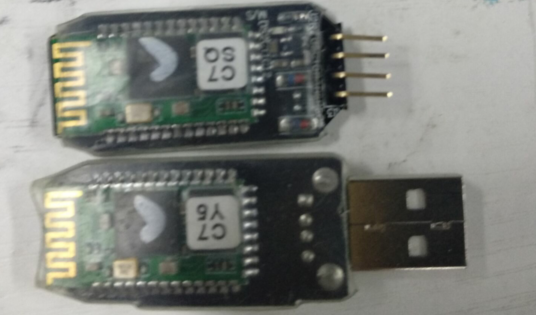2、有线蓝牙串口：每队蓝牙串口后面都有排插，可以蓝牙间直接通过导线直接连接。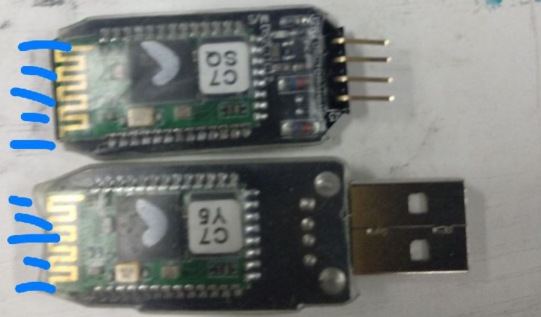3、蓝牙串口硬件及程序部分

``````UART_Init(UART_4,115200);   //蓝牙或串口初始化
``````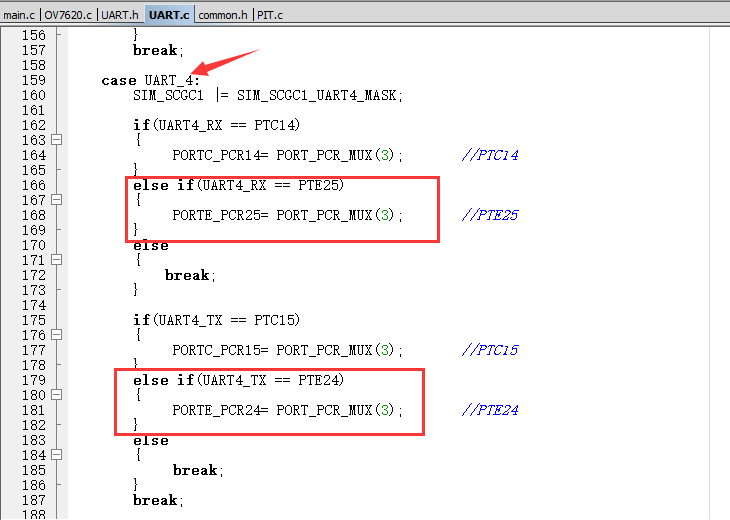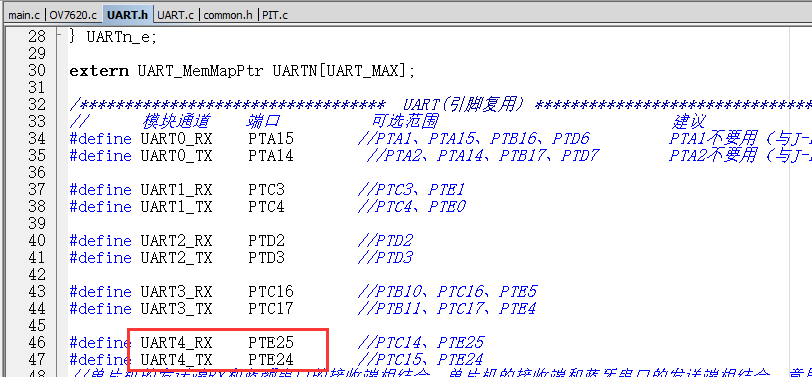## 单工，半双工和全双工

1.单工数据传输只支持数据在一个方向上传输；在同一时间只有一方能接受或发送信息，不能实现双向通信，举例：电视，广播。

2.半双工数据传输允许数据在两个方向上传输,但是,在某一时刻,只允许数据在一个方向上传输,它实际上是一种切换方向的单工通信；在同一时间只可以有一方接受或发送信息，可以实现双向通信。举例：对讲机。3.全双工数据通信允许数据同时在两个方向上传输,因此,全双工通信是两个单工通信方式的结合,它要求发送设备和接收设备都有独立的接收和发送能力；在同一时间可以同时接受和发送信息，实现双向通信，举例：电话通信。

4.网卡的全双工（Full Duplex）是指网卡在发送数据的同时也能够接收数据,两者同步进行,这好像我们平时打电话一样,说话的同时也能够听到对方的声音.目前的网卡一般都支持全双工.

## AT命令

AT命令有两种解释一种是调制解调器命令语言，另一种是Windows中的计划任务命令行。

## 线性CCD

CCD传感器:电荷耦合器件图像传感器CCD（Charge Coupled Device）
CCD传感器是一种新型光电转换器件，它能存储由光产生的信号电荷

## COM口

COM口( cluster communication port )即串行通讯端口，简称串口。微机上的串口通常是9针，也有25针的接口，最大速率115200bps。通常用于连接鼠标（串口）及通讯设备（如连接外置式调制解调器进行数据通讯或一些工厂的数控机接口）等。一般主板外部只有一个串口，机箱后面和并口一起的那个九孔输出端（梯形），就是COM1口，COM2口一般要从主板上插针引出。并口是最长的那个梯形口。

## 摄像头调试助手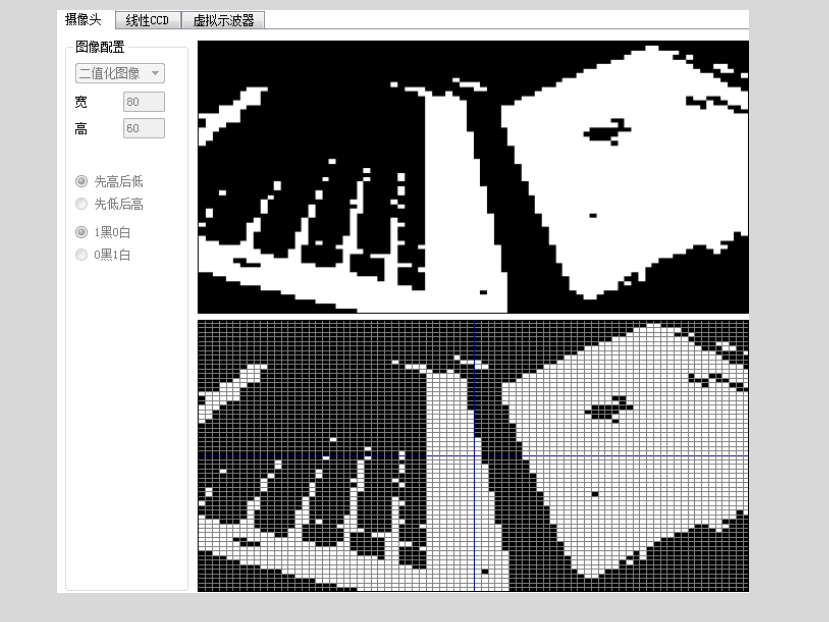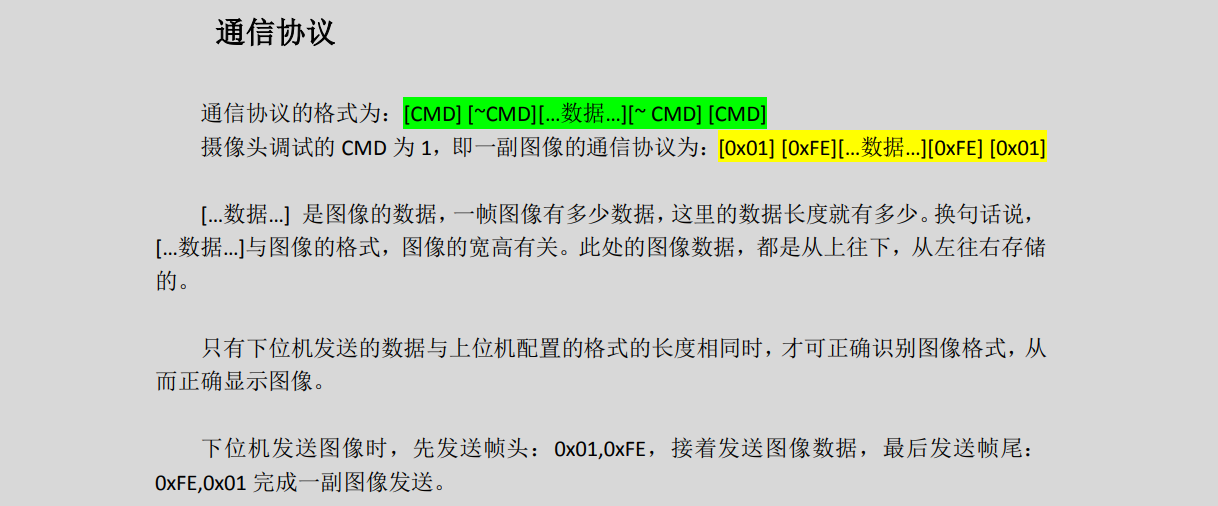## 线性 CCD 调试助手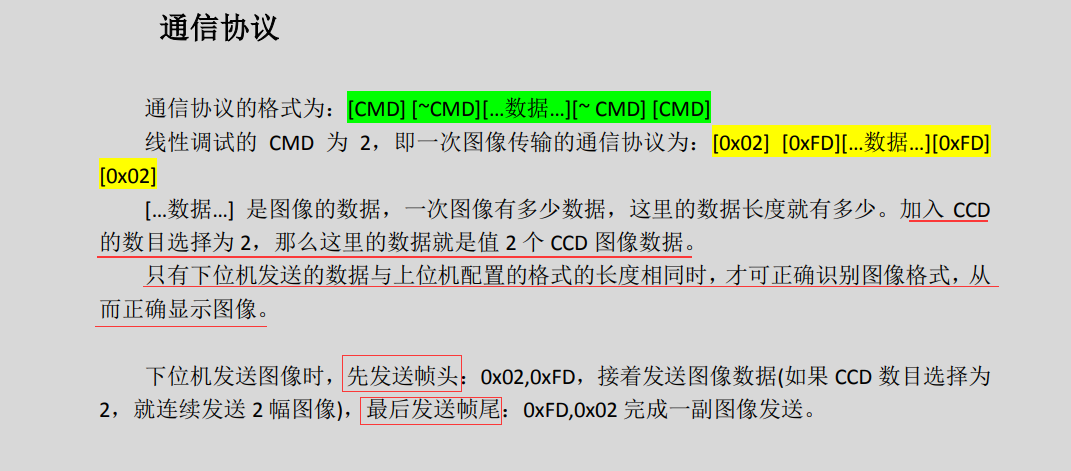## 虚拟示波器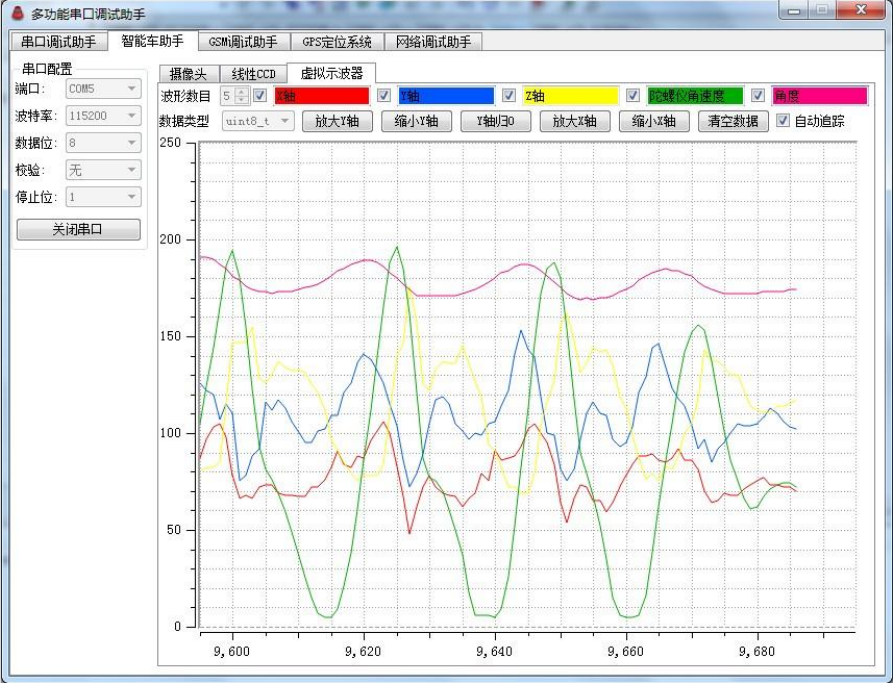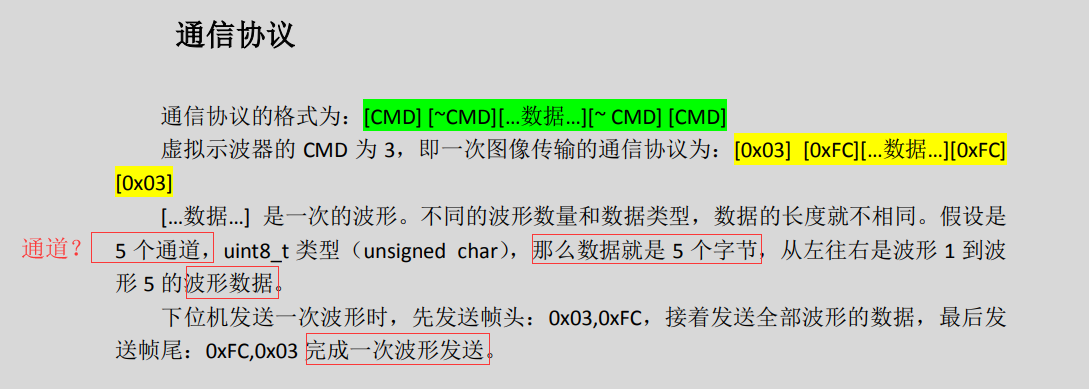## PDB

PDB 可以为内部或外部触发源提供可控制的延时，或者为 ADC 的硬件触发或产生 DAC 提供可编程的间隔。这样就可以为 ADC 转换和 DAC 输出的完成提供精确的时间。PDB 模块还可以提供脉冲输出，就行 CMP 模块 中的采样窗口一样。

## 弹性定时器(FlexTimer，FTM)

FTM 模块只有一个时钟域就是系统时钟。

### 1、计数器同步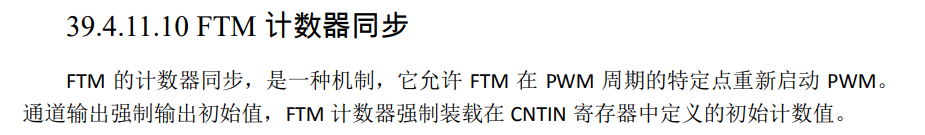## 指针

### void 指针类型

【例如】：

`````` float *p1; //声明 p1 为浮点型指针
int *p2; //声明 p2 为整型指针
p1 = (float *)p2;//强制转换整型指针 p2 为浮点型指针值给 p1 赋值
而 void *则不同，任何类型的指针都可以直接赋值给它，无需进行强制类型转
``````

``````void *p1; //声明 p1 无类型指针
int *p2; //声明 p2 为整型指针
p1 = p2; //用整型指针 p2 的值给 p1 直接赋值
``````

## 结构体

1）结构体的说明和结构体变量的定义
【例如】定义一个名为 student 的结构体变量类型

``````struct student //定义一个名为student的结构体变量类型
{ char name; //成员变量“name”为字符型数组
char class; //成员变量“class”为字符型数组
int age; //成员变量“age”为整型
};
``````

struct student s1; //声明s1为“student”类型的结构体变量
【例如】定义一个名为 student 的结构体变量类型，同时声明 s1 为一个“student”类型的

``````struct student //定义一个名为student的结构体变量类型
{ char name; //成员变量“name”为字符型数组
char class; //成员变量“class”为字符型数组
int age; //成员变量“age”为整型
}s1; //声明s1为“student”类型的结构体变量
``````

2）结构体变量的使用

【例如】：

``````s1.age=18; //将数据 18 赋给 s1.age（理解为学生 s1 的年龄为 18）
``````

3）结构体指针

``````struct student *Pstudent; //声明 Pstudent 为一个“student”类型指针
``````

``````strcpy(Pstudent->name,"LiuYuZhang");
Pstudent->age=18;
``````

``````Pstudent=(struct student*)malloc(sizeof (struct student));
sizeof（struct student）自动求取 student 结构体的字节长度，
malloc（）函数定义了一个大小为结构体长度的内存区域，然后将其地址作为结构体指针返回。
``````

## 通用异步接收器/发送器(UART)

UART 允许与外围设备及其他 CPU 进行异步串行通信。

2019-04-08 18:57:38 pro1515151515 阅读数 371
• ###### 单片机控制第一个外设-LED灯-第1季第6部分

本课程是《朱有鹏老师单片机完全学习系列课程》第1季第6个课程，主要讲解LED的工作原理和开发板原理图、实践编程等，通过学习目的是让大家学会给单片机编程控制LED灯，并且为进一步学习其他外设打好基础。

4000 人正在学习 去看看 朱有鹏

# C51代码（矩阵键盘连接P3口）

1.定义全局变量

``````uchar key_TTL;
uint keys_val=0;
uint last_keys_val=0;
uchar max_TTL=3;``````

2.在key_spy()函数中添加按键功能

``````void key_spy()
{
uint temp=0;
//双循环扫描法 + 译码
uchar k,l;
for(l=0;l<4;l++){
P3=~(0x01<<l);
for(k=0;k<4;k++){
if((P3&(0x10<<k))==0) key_TTL[k+l*4]+=2;
}
}
//TTL去抖
for(k=0;k<16;k++){
if(key_TTL[k]>0)key_TTL[k]--;
if(key_TTL[k]>=max_TTL){
key_TTL[k]--;
temp=temp|(1<<k);
}
}
keys_val=temp;
//按键功能
if(keys_val!=last_keys_val){
if(keys_val==((1<<0))){
//TODO:按下了0号键

}
else if(keys_val==((1<<3)|(1<<4)|(1<<5))){
//TODO:同时按下了3号键、4号键、5号键

}
//TODO:加入更多else if,以扩充矩阵键盘功能

}
last_keys_val=keys_val;
}``````

3.主循环中添加key_spy()函数

``````void main()
{

while(1)
{
key_spy();
//TODO:主循环的其他事物

}
}
``````

# 原理介绍

## 软件去抖的原理

key_spy()函数是检测矩阵键盘用的，放在主循环里就每隔一段时间检测一次。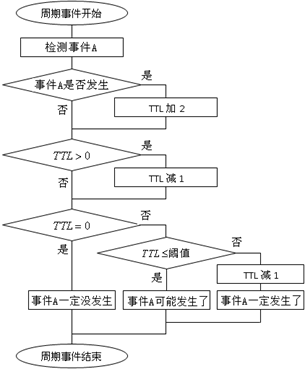## 组合键的原理

### 线反转法

``````void key_spy1()
{
//线反转法
uchar flag=1,key,k;
P3=0xf0;
key=P3&0xf0;
P3=0x0f;
key|=(P3&0x0f);
switch(key)
{
case 0xee: key_TTL+=2; break;
case 0xde: key_TTL+=2; break;
case 0xbe: key_TTL+=2; break;
case 0x7e: key_TTL+=2; break;
case 0xed: key_TTL+=2; break;
case 0xdd: key_TTL+=2; break;
case 0xbd: key_TTL+=2; break;
case 0x7d: key_TTL+=2; break;
case 0xeb: key_TTL+=2; break;
case 0xdb: key_TTL+=2; break;
case 0xbb: key_TTL+=2; break;
case 0x7b: key_TTL+=2; break;
case 0xe7: key_TTL+=2; break;
case 0xd7: key_TTL+=2; break;
case 0xb7: key_TTL+=2; break;
case 0x77: key_TTL+=2; break;
default:break;
}

//check TTL
for(k=0;k<16;k++)
{
if(key_TTL[k]>0)key_TTL[k]--;
if(key_TTL[k]>=max_TTL)//>=改为=，就能自动连点
{
key_TTL[k]--;
key_val=k+1;
flag=0;
}
}
if(flag)key_val=0;//全局变量key_val即为键值
}``````

### 逐行扫描法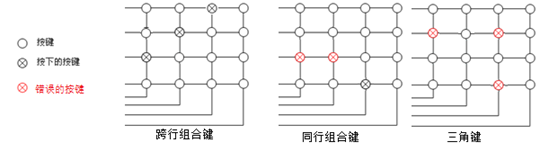``````void key_spy2()
{
//逐行扫描法
uchar flag=1,k;
P3=0xfe;
switch(P3)
{
case 0xee: key_TTL+=2; break;
case 0xde: key_TTL+=2; break;
case 0xbe: key_TTL+=2; break;
case 0x7e: key_TTL+=2; break;
}
P3=0xfd;
switch(P3)
{
case 0xed: key_TTL+=2; break;
case 0xdd: key_TTL+=2; break;
case 0xbd: key_TTL+=2; break;
case 0x7d: key_TTL+=2; break;
}
P3=0xfb;
switch(P3)
{
case 0xeb: key_TTL+=2; break;
case 0xdb: key_TTL+=2; break;
case 0xbb: key_TTL+=2; break;
case 0x7b: key_TTL+=2; break;
}
P3=0xf7;
switch(P3)
{
case 0xe7: key_TTL+=2; break;
case 0xd7: key_TTL+=2; break;
case 0xb7: key_TTL+=2; break;
case 0x77: key_TTL+=2; break;
}

//check TTL
for(k=0;k<16;k++)
{
if(key_TTL[k]>0)key_TTL[k]--;
if(key_TTL[k]>=max_TTL)//>=改为=，就能自动连点
{
key_TTL[k]--;
key_val=k+1;
flag=0;
}
}
if(flag)key_val=0;//全局变量key_val即为键值
}``````

### 双循环扫描法

``````void key_spy3()
{
//双循环扫描法
uchar flag=1,k;
P3=0xfe;P3=0xfe;//高四位设为输入
OLED_write_bchar(0,0,P3);
if((P3&0x80)==0) key_TTL+=2;
if((P3&0x40)==0) key_TTL+=2;
if((P3&0x20)==0) key_TTL+=2;
if((P3&0x10)==0) key_TTL+=2;
P3=0xfd;P3=0xfd;//高四位设为输入
if((P3&0x80)==0) key_TTL+=2;
if((P3&0x40)==0) key_TTL+=2;
if((P3&0x20)==0) key_TTL+=2;
if((P3&0x10)==0) key_TTL+=2;
P3=0xfb;P3=0xfb;//高四位设为输入
if((P3&0x80)==0) key_TTL+=2;
if((P3&0x40)==0) key_TTL+=2;
if((P3&0x20)==0) key_TTL+=2;
if((P3&0x10)==0) key_TTL+=2;
P3=0xf7;P3=0xf7;//高四位设为输入
if((P3&0x80)==0) key_TTL+=2;
if((P3&0x40)==0) key_TTL+=2;
if((P3&0x20)==0) key_TTL+=2;
if((P3&0x10)==0) key_TTL+=2;

//check TTL
for(k=0;k<16;k++)
{
if(key_TTL[k]>0)key_TTL[k]--;
if(key_TTL[k]>=max_TTL)//>=改为=，就能自动连点
{
key_TTL[k]--;
key_val=k+1;
flag=0;
}
}
if(flag)key_val=0;//全局变量key_val即为键值
}``````